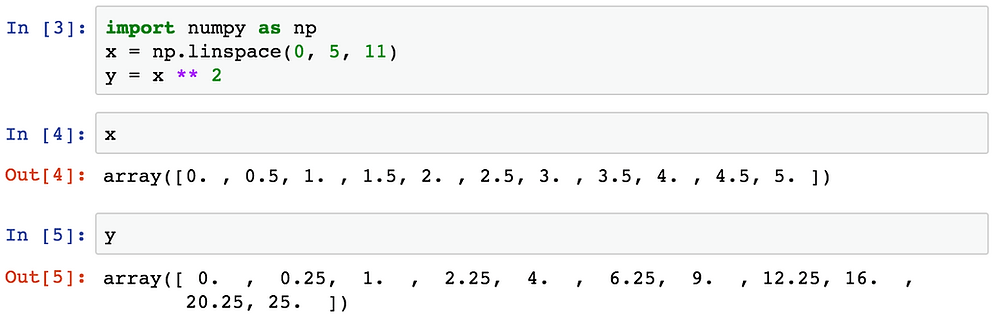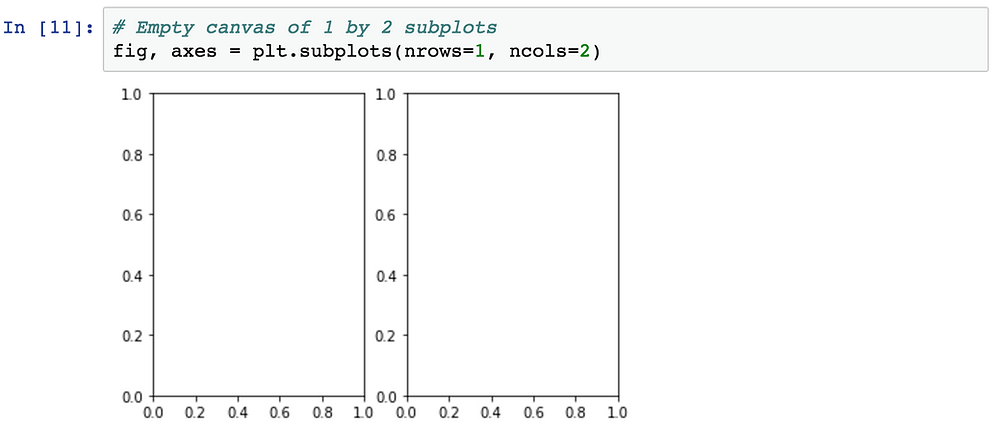Search

# Exploratory Data Analysis In Data Science | Data Science Homework Help In USA | Realcode4you

We are offering Data Science Homework Help, Assignment Help, Project Help, etc. in mote then 10 countries like USA, Canada, etc.

In this blog we will learn the exploratory in data Science.

1. Introduction

2. Matplotlib Object Oriented Method

3. Legends, labels and titles

4. Plot range

5. Special Plot Types

6. Exercises

### 1. Introduction

Matplotlib is the "grandfather" library of data visualization with Python. It was created by John Hunter. He created it to try to replicate MatLab's (another programming language) plotting capabilities in Python. So if you happen to be familiar with matlab, matplotlib will feel natural to you.

It is an excellent 2D and 3D graphics library for generating scientific figures.

Matplotlib allows you to create reproducible figures programmatically. Let's learn how to use it! Before continuing this lecture, I encourage you just to explore the official Matplotlib web page: http://matplotlib.org/

1.1 . Importing

Import the matplotlib.pyplot module under the name plt (the tidy way):

>>> import matplotlib.pyplot as plt

You'll also need to use this line to see plots in the notebook:

>>> %matplotlib inline

That line is only for jupyter notebooks, if you are using another editor, you'll use: plt.show() at the end of all your plotting commands to have the figure pop up in another window

1.2 . Basic example

Let's walk through a very simple example using two numpy arrays. You can also use lists, but most likely you'll be passing numpy arrays or pandas columns (which essentially also behave like arrays).1.3 . Basic Matplotlib Commands

We can create a very simple line plot using the following ( I encourage you to pause and use Shift+Tab along the way to check out the document strings for the functions we are using).1.4 . Creating Multiplots on Same Canvas### 2. Matplotlib Object Oriented Method

Now that we've seen the basics, let's break it all down with a more formal introduction of Matplotlib's Object Oriented API. This means we will instantiate figure objects and then call methods or attributes from that object.

2.1 . Introduction to the Object Oriented Method

The main idea in using the more formal Object Oriented method is to create figure objects and then just call methods or attributes off of that object. This approach is nicer when dealing with a canvas that has multiple plots on it.

To begin we create a figure instance. Then we can add axes to that figure:Code is a little more complicated, but the advantage is that we now have full control of where the plot axes are placed, and we can easily add more than one axis to the figure:2.2 . subplots()

The plt.subplots() object will act as a more automatic axis manager.Then you can specify the number of rows and columns when creating the subplots() object:A common issue with matplolib is overlapping subplots or figures. We ca use fig.tight_layout() or plt.tight_layout() method, which automatically adjusts the positions of the axes on the figure canvas so that there is no overlapping content2.3 . Figure size, aspect ratio and DPI

Matplotlib allows the aspect ratio, DPI and figure size to be specified when the Figure object is created. You can use the figsize and dpi keyword arguments.

figsize is a tuple of the width and height of the figure in inchesdpi is the dots-per-inch (pixel per inch).

For example:2.4 . Saving figures

Matplotlib can generate high-quality output in a number formats, including PNG, JPG, EPS, SVG, PGF and PDF.

To save a figure to a file we can use the savefig method in the Figure class:

>>>fig.savefig("fig1")

### 3. Legends, labels and titles

Now that we have covered the basics of how to create a figure canvas and add axes instances to the canvas, let's look at how decorate a figure with titles, axis labels, and legends.

3.1 . Figure titles

A title can be added to each axis instance in a figure. To set the title, use the set_title method in the axes instance:

>>> ax.set_title("title");

3.2 . Axis labels

Similarly, with the methods set_xlabel and set_ylabel, we can set the labels of the X and Y axes:

>>> ax.set_xlabel("x")

>>> ax.set_ylabel("y");

3.3 . Legends

You can use the label="label text" keyword argument when plots or other objects are added to the figure, and then using the legend method without arguments to add the legend to the figure:### 4. Plot range

We can configure the ranges of the axes using the set_ylim and set_xlim methods in the axis object, or axis('tight') for automatically getting "tightly fitted" axes ranges:### 5. Special Plot Types

There are many specialized plots we can create, such as barplots, histograms, scatter plots, and much more. Most of these type of plots we will actually create using seaborn, a statistical plotting library for Python. But here are a few examples of these type of plots:Other services which is offered by us:

<Realcode4you> Assignment Help

<Realcode4you> Web Assignment Help

Thanks for reading <realcode4you> blog, if you need any help or want to help in python data science exploratory than contact us at here.